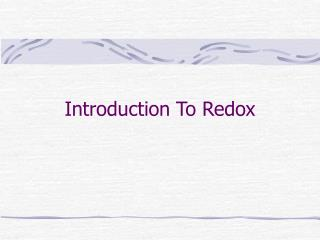Download PresentationIntroduction To Redox

# Introduction To Redox

Download Presentation## Introduction To Redox

- - - - - - - - - - - - - - - - - - - - - - - - - - - E N D - - - - - - - - - - - - - - - - - - - - - - - - - - -
##### Presentation Transcript

1. Introduction To Redox

2. Types of Reaction • Oxidation-Reduction called Redox • Ionic compounds are formed through the transfer of electrons. • An Oxidation-reduction reaction involves the transfer of electrons. • We need a way of keeping track.

3. Oxidation States • A way of keeping track of the electrons. • Not necessarily true of what is in nature, but it works. • need the rules for assigning (memorize). • The oxidation state of elements in their standard states is zero. • Oxidation state for monoatomic ions are the same as their charge.

4. Oxidation states • Oxygen is assigned an oxidation state of -2 in its covalent compounds except as a peroxide. • In compounds with nonmetals hydrogen is assigned the oxidation state +1. • In its compounds fluorine is always –1. • The sum of the oxidation states must be zero in compounds or equal the charge of the ion.

5. Oxidation States • Assign the oxidation states to each element in the following. • CO2 • NO3- • H2SO4 • Fe2O3 • Fe3O4

6. Oxidation-Reduction • Transfer electrons, so the oxidation states change. • Na + 2Cl2® 2NaCl • CH4 + 2O2® CO2 + 2H2O • Oxidation is the loss of electrons. • Reduction is the gain of electrons. • OIL RIG • LEO GER

7. Oxidation-Reduction • Oxidation means an increase in oxidation state - lose electrons. • Reduction means a decrease in oxidation state - gain electrons. • The substance that is oxidized is called the reducing agent. • The substance that is reduced is called the oxidizing agent.

8. Redox Reactions

9. Agents • Oxidizing agent gets reduced. • Gains electrons. • More negative oxidation state. • Reducing agent gets oxidized. • Loses electrons. • More positive oxidation state.

10. Identify the • Oxidizing agent • Reducing agent • Substance oxidized • Substance reduced • in the following reactions • Fe(s) + O2(g) ® Fe2O3(s) • Fe2O3(s)+ 3 CO(g) ® 2 Fe(l) + 3 CO2(g) • SO3- + H+ + MnO4- ® SO4- + H2O + Mn+2

11. Half-Reactions • All redox reactions can be thought of as happening in two halves. • One produces electrons - Oxidation half. • The other requires electrons - Reduction half. • Write the half reactions for the following. • Na + Cl2® Na+ + Cl- • SO3- + H+ + MnO4- ® SO4- + H2O + Mn+2

12. Balancing Redox Equations • In aqueous solutions the key is the number of electrons produced must be the same as those required. • For reactions in acidic solution an 8 step procedure. • Write separate half reactions • For each half reaction balance all reactants except H and O • Balance O using H2O

13. Acidic Solution • Balance H using H+ • Balance charge using e- • Multiply equations to make electrons equal • Add equations and cancel identical species • Check that charges and elements are balanced.

14. Practice • The following reactions occur in aqueous solution. Balance them • Cr(OH)3 + OCl- + OH-® CrO4-2 + Cl- + H2O • MnO4- + Fe+2® Mn+2 + Fe+3 • Cu + NO3-® Cu+2+ NO(g) • Pb + PbO2 + SO4-2® PbSO4 • Mn+2+ NaBiO3® Bi+3+ MnO4-

15. Now for a tough one • Fe(CN)6-4 + MnO4-® Mn+2+ Fe+3 + CO2 + NO3-

16. Basic Solution • Do everything you would with acid, but add one more step. • Add enough OH- to both sides to neutralize the H+ • CrI3 + Cl2® CrO4-+ IO4- + Cl- • Fe(OH)2 + H2O2® Fe(OH)-

17. Redox Titrations • Same as any other titration. • the permanganate ion is used often because it is its own indicator. MnO4- is purple, Mn+2 is colorless. When reaction solution remains clear, MnO4- is gone. • Chromate ion is also useful, but color change, orangish yellow to green, is harder to detect.

18. Example • The iron content of iron ore can be determined by titration with standard KMnO4 solution. The iron ore is dissolved in excess HCl, and the iron reduced to Fe+2 ions. This solution is then titrated with KMnO4 solution, producing Fe+3 and Mn+2 ions in acidic solution. If it requires 41.95 mL of 0.205 M KMnO4 to titrate a solution made with 0.6128 g of iron ore, what percent of the ore was iron?